Next: Signal and noise PEFs Up: Multiple attenuation: Theory and Previous: Introduction

# Filter estimation

The key assumption of the proposed multiple attenuation technique is that primaries and multiples have different multidimensional spectra that PEFs can approximate Claerbout (1992); Spitz (1999). These filters can be interpreted as multidimensional dip filters. The estimation of these filters is based on the spatial predictability of seismic events. Therefore, PEFs for primaries and multiples are needed prior to the subtraction step. This section describes how these filters are estimated.

The PEFs used here are time-space domain non-stationary filters to cope with the variability of seismic data with time, offset and shot position. Implementing non-stationary filters is not an easy task. A possible solution is to break-up the dataset into patches and estimate a filter for each patch. However, reassembling these patches create edge effects in the overlapping zones. Alternatively, non-stationary convolution or combination can be used to estimate one filter per data point Margrave (1998) or more realistically, per micro-patch Crawley (2000). A micro-patch is made of a succession of data points that share the same filter. With this technique, the filters can vary smoothly across the dataset while leaving almost no edge effect.

A detailed description of the non-stationary filters is given in Appendix. Here I present the most important steps of the filter estimation procedure only. Callingthe non-stationary combination matrix Margrave (1998) with the data vectorfrom which we want to estimate the filters andthe unknown PEFs coefficients, one way to estimate the PEFs is to minimize the length of residual vectorsClaerbout and Fomel (2002):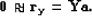(45)
This last expression is also called fitting goal. By definition of the PEFs, the first coefficient is always one (Figure). To take this requirement into account, equation () becomes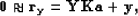(46)
where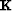is a masking operator that forces the first coefficient of the PEFs to be one.

If one filter is used per data point, the matrix of unknown coefficientscan be enormous, making the problem very underdetermined. This difficulty can be overcome in two ways. Firstly, the filter is kept constant inside a micro-patch. Secondly, a smoothing operatoris introduced to penalize strong variations between neighboring filters. Both strategies are considered here. Introducing the Laplacian operator, equation () is augmented as follows: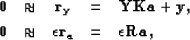(47)
whereis a trade-off parameter between coefficient estimation and filter smoothing. The Laplacian operator smoothes along two (i.e., time/offset) or three axes (i.e., time/offset/shot) depending on the PEF's dimensions. A least-squares estimate of the PEF's coefficients leads to the following objective function: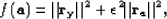(48)
whereis estimated iteratively with the conjugate-gradient method andis chosen by trial and error. Note that the Huber norm of Chapteris not used here.

The amplitudes of seismic data vary across offset, shot and time. Large amplitude variations can be troublesome with least-squares inversion because they tend to bias the final result Claerbout (1992) by ignoring or over-fitting some areas of the data. Therefore, it is important to make sure that these amplitude variations do not affect our processing. One solution is to apply a weight to the data prior to the inversion like Automatic Gain Control (AGC) or a geometrical spreading correction. Alternatively, a weight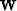can be incorporated inside the fitting goals in equation ():(49)
This weight can be interpreted as a change of norm consistent with the data, similar to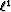norm. In this Chapter,is obtained by computing for each shot gather(50)
where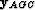is the data vector with AGC applied. If division by zero occurs, the weight is set to zero. This weight can also incorporate a mute zone where no data are present. Thanks to the helical boundary conditions Claerbout (1998); Mersereau and Dudgeon (1974), the PEFs can have any dimension. In this Chapter, I use 2-D (time/offset) and 3-D (time/offset/shot position)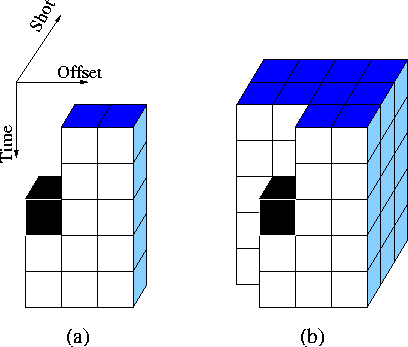pef
Figure 1
prediction-error filters in (a) 2-D and (b) 3-D. The first coefficient in black is always one.filters (Figure) and demonstrate that 3-D filters lead to the best noise attenuation results. When 2-D filters are used, the multiple attenuation is performed on one shot gather at a time. When 3-D filters are used, the multiple attenuation is performed on one macro-gather at a time. A macro-gather is a cube made of adjacent shots with all the offsets and time samples. There is an overlapping zone of five shot gathers between neighboring macro-patches. When the multiple attenuation is done, the macro-gathers are reassembled to form the final result. The next section describes how to choose the modelin equation () when PEFs for multiples and primaries are estimated.Next: Signal and noise PEFs Up: Multiple attenuation: Theory and Previous: Introduction
Stanford Exploration Project
5/5/2005